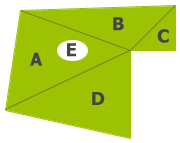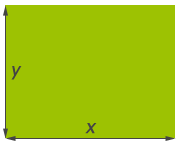Mobile nav

This calculator helps with tallying the amount of turf required for simple or complex shaped areas.

To calculate the area of an irregular shaped plot, divide the area into regular shapes and then add or subtract them, as required.For example, the plot shown left breaks down into four triangles and an ellipse shaped flower bed, so the area is:

Area = A + B + C + D - E

The individual areas A to E are obtained using the calculators below and accumulate in the table on the right. See example.

To use the turf calculator:

1. Make a plan of your area and divide it into easily measurable sections – rectangles, triangles, ellipses, circles etc – for both the turf area and any beds or patios;
2. for each section, enter the x, y, z measurements for its shape in the calculators below, then click Add to Total if it is to be turfed, or Subtract from Total if it is to be left unturfed;
3. the sections will appear in the table on the right as you work, with a tally of the area to turf;
4. set the measurement units you used on the right – metres, yards or feet; this converts the total area to square metres, rounded up to the nearest unit;
5. click Get Price to get a quote for this quantity or to place an order.

Squares and RectanglesShape:

(either order)

Area =

Triangles(any order)

Area =

Circles and Ellipses

Note that an oval shape may not be a true ellipse! This calculator is accurate for ellipses but should approximate other squashed circles quite well.

Shape:(either order)

Area =

Other shapes

Divide the area into triangles by measuring across opposite corners and then use the calculators above.